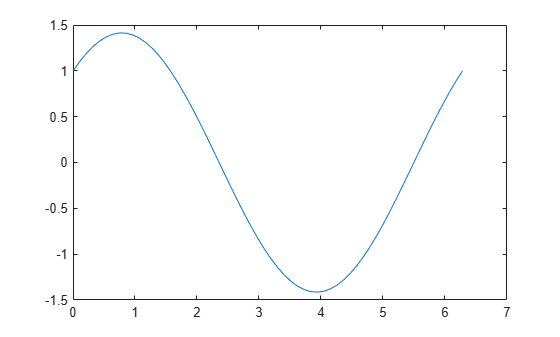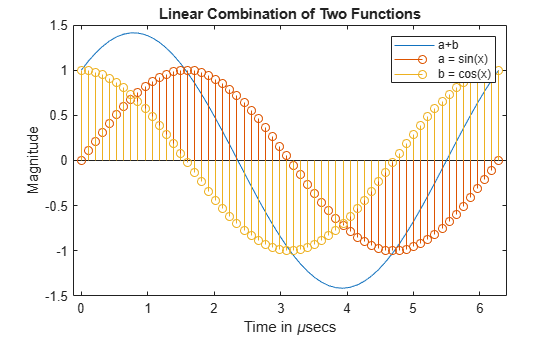# Combine Line and Stem Plots

This example shows how to combine a line plot and two stem plots. Then, it shows how to add a title, axis labels, and a legend.

Create the data and plot a line.

```x = linspace(0,2*pi,60); a = sin(x); b = cos(x); plot(x,a+b)```Add two stem plots to the axes. Prevent new plots from replacing existing plots using `hold on`.

```hold on stem(x,a) stem(x,b) hold off```Add a title, axis labels, and a legend. Specify the legend descriptions in the order that you create the plots.

```title('Linear Combination of Two Functions') xlabel('Time in \musecs') ylabel('Magnitude') legend('a+b','a = sin(x)','b = cos(x)')```